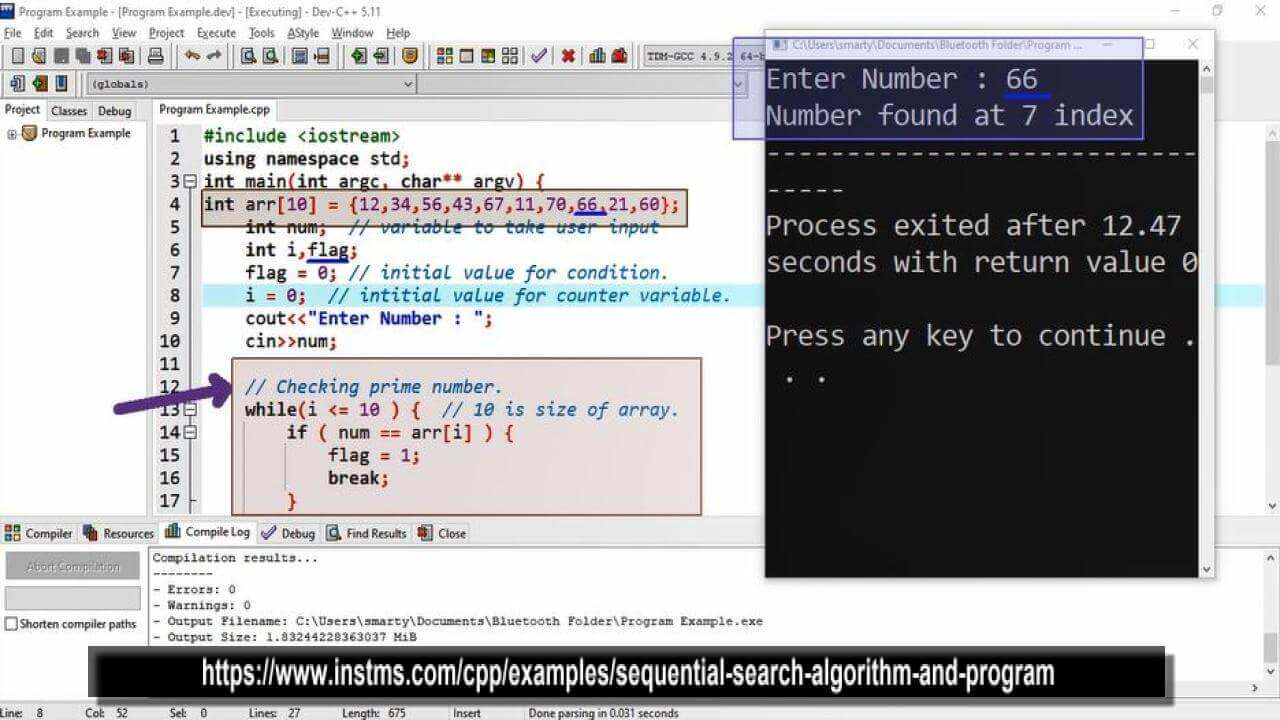# Sequential Search Algorithm And Program using CPP

## Source Code

#include <iostream>

using namespace std;

int main(int argc, char** argv) {

int arr = {12,34,56,43,67,11,70,66,21,60};
int num;  // variable to take user input
int i,flag;
flag = 0; // initial value for condition.
i = 0;  // intitial value for counter variable.

cout<<"Enter Number : ";
cin>>num;

// Checking prime number.
while(i <= 10 ) {  // 10 is size of array.
if ( num == arr[i] ) {
flag = 1;
break;
}
i++;
}

if(flag == 0) {
} else {
cout<<"Number found at "<<i<<" index";
}
return 0;
}

##Working

An array of size 10 is created and initialized with some random data values. Then three variables are declared and initialized with required values as given below.

 Sr. Name Initial Value Purpose 1 num Taken by user as input Value of this variable will match from array 2 flag 0 Will used as condition. Change to 1if particular condition will true. 3 i 0 Counter variable for iteration

After declaration we will take input from user and use a loop for matching the input values with given array/data set. If any value of array match with provided input then we will terminate iteration using break statement and change value of flag variable with 1.

We can use for or do while loop in place of while loop